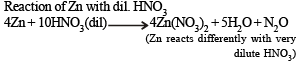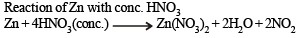Courses

# Test: 35 Year JEE Previous Year Questions: The p-Block Elements

## 41 Questions MCQ Test Chemistry 35 Years JEE Main & Advanced Past year Papers | Test: 35 Year JEE Previous Year Questions: The p-Block Elements

Description
This mock test of Test: 35 Year JEE Previous Year Questions: The p-Block Elements for JEE helps you for every JEE entrance exam. This contains 41 Multiple Choice Questions for JEE Test: 35 Year JEE Previous Year Questions: The p-Block Elements (mcq) to study with solutions a complete question bank. The solved questions answers in this Test: 35 Year JEE Previous Year Questions: The p-Block Elements quiz give you a good mix of easy questions and tough questions. JEE students definitely take this Test: 35 Year JEE Previous Year Questions: The p-Block Elements exercise for a better result in the exam. You can find other Test: 35 Year JEE Previous Year Questions: The p-Block Elements extra questions, long questions & short questions for JEE on EduRev as well by searching above.
QUESTION: 1

### Alum helps in purifying water by

Solution:

Alum furnishes Al3+ ions which bring about coagulation of negatively charged clay particles, bacteria etc.

QUESTION: 2

### In XeF2, XeF4, XeF6 the number of lone pairs on Xe are respectively

Solution:

In the formation of  XeF2, sp3d hybridisation occurs which gives the molecule a trigonal bipyramidal structure.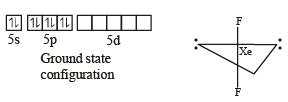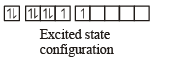In the formation of XeF4, sp3d2 hybridization occurs which gives the molecule an octahedral structure.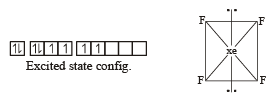In the formation of XeF6, sp3d3 hybridization occurs which gives the molecule a pentagonal bipyramidal structure.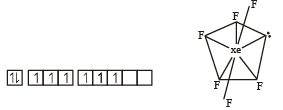QUESTION: 3

### In case of nitrogen, NCl3 is possible but not NCl5 while in case of phosphorous, PCl3 as well as PCl5 are possible. It is due to

Solution: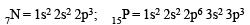NOTE :  In phosphorous the 3d- orbitals are available.
Hence phosphorous can from pentahalides also but nitrogen can not form pentahalide due to absence of dorbitals

QUESTION: 4

Which of the following statements is true?

Solution:
QUESTION: 5

Number of sigma bonds in P4O10 is

Solution: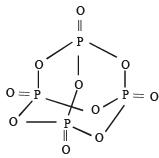QUESTION: 6

Oxidation number of Cl in CaOCl2 (bleaching power) is:

Solution: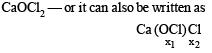hence oxidation no of Cl in OCl– is
–2 + x2 = –1
x2 = 2–1 = +1
now oxidation no. of another Cl is –1 as it is present as Cl.

QUESTION: 7

What may be expected to happen when phosphine gas is mixed with chlorine gas ?

Solution:

On mixing phosphine with chlorine gas PCl5 and HCl forms. The mixture cools down.
PH3 + 4Cl2 –––––→  PCl5 + 3HCl

QUESTION: 8

Concentrated hydrochlor ic acid when kept in open air sometimes produces a cloud of white fumes. The explanation for it is that

Solution: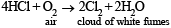QUESTION: 9

Graphite is a soft solid lubricant extremely difficult to melt. The reason for this anomalous behaviour is that graphite

Solution:

In graphite, carbon is sp2 hybridized. Each carbon is thus linked to three other carbon atoms forming hexagonal rings. Since only three electrons of each carbon are used in making hexagonal ring, fourth electron of each carbon is free to move. This makes graphite a good conductors of heat and electricity.
Further graphite has a two dimensional sheet like structure. These various sheets are held together by weak van der Waal’s force of attraction. due to these weak forces of attraction, one layer can slip over the other. This makes graphite soft and a good lubricating agent.

QUESTION: 10

Glass is a

Solution:

Glass is a translucent or transparent amorphous supercooled solid solution or we can say super cooled liquid of silicates and borats having a general formula R2O. MO. 6 SiO2. where R = Na or K and M = Ca, Ba, Zn or Pb.

QUESTION: 11

Which one of the following substan ces has the highest proton affinity ?

Solution:

Among  the given compounds, the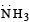is most basic. Hence has highest proton affinity

QUESTION: 12

For making good quality mirrors, plates of float glass are used. These are obtained by floating molten glass over a liquid metal which does not solidify before glass. The metal used can be

Solution:

It is mer cury because it exists as liquid at room temperature.

QUESTION: 13

Which among the following factors is the most important in making fluorine the strongest oxidizing halogen ?

Solution:

Fluorine is the strongest oxidizing agent due to low heat of dissociation and high heat of hydration which compensate the lower value of electron affinity.

QUESTION: 14

Which one of the following statement regarding helium is incorrect ?

Solution:

Property of He -It is used in weather balloons and tires of airplanes as He is non inflammable. He is heavier than H. So the correct option is c.

QUESTION: 15

Beyllium and aluminium exhibit many properties which are similar. But, the two elements differ in

Solution:

The maximum valency of beryllium is +2 while that of aluminium is +3.

QUESTION: 16

Aluminium chloride exists as dimer, Al2Cl6 in solid state as well as in solution of non-polar solvents such as benzene.
When dissolved in water, it gives

Solution: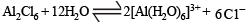QUESTION: 17

Excess of KI reacts with CuSO4 solution and then Na2S2O3 solution is added to it. Which of the statements is incorrect for this reaction ?

Solution: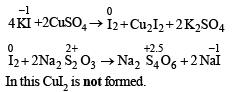QUESTION: 18

The number of hydrogen atom(s) attached to phosphorus atom in hypophosphorous acid is

Solution:

Hypophosphorous acid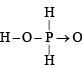QUESTION: 19

The correct order of the thermal stability of hydrogen halides (H – X) is

Solution:

The H–X bond strength decreases from HF to HI. i.e.
HF > HCl > HBr > HI. Thus HF is most stable while HI is least stable. This is evident from their decomposition reaction: HF and HCl are stable upto 1473K, HBr decreases slightly and HI dissociates considerably at 713K. The decreasing stability of the hydrogen halide is also reflected in the values of dissociation energy of the H–X bond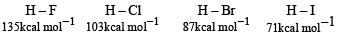QUESTION: 20

Heating an aqueous solution of alumin ium chloride to dryness will give

Solution:

The solution of aluminium chloride in water is acidic due to hydrolysis.

AlCl3 + 3H2O –––––→ Al(OH)3 +  3HCl.
On heating  it till dryness Al(OH)3 is converted into Al2O3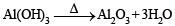QUESTION: 21

In silicon dioxide

Solution:

In SiO2 (quartz), each of O-atom is shared between two SiO44– tetrahedra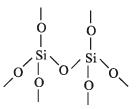QUESTION: 22

The structure of diborane (B2H6) contains

Solution:

In diborane structure B2H6 there are two 2c-2e bonds and two 3c–2e bonds (see structure of diborane).
Structure of B2H6 :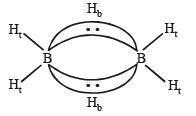QUESTION: 23

Which of the following statements is true?

Solution:

The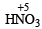is stronger than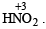The more the oxidation state of N, the more is the acid character.

QUESTION: 24

The increasing order of the first ionization enthalpies of the elements B, P, S and F (Lowest first) is

Solution:

The correct order of ionisation enthalpies is

F > P > S > B

NOTE : On moving along a period ionization enthalapy increases from left to right and decreases from top to bottom in a group. But this trend breaks up in case of atom having  fully or half filled stable orbitals.
In this case P has a stable half filled electronic configuration hence its ionisation enthalapy is greater in comparision to S. Hence the correct order is

B <  S < P < F.

QUESTION: 25

What products are expected from the disproportionation reaction of hypochlorous acid?

Solution:

During disproportionation same compound undergo simultaneous oxidation and reduction.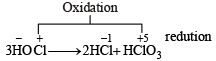QUESTION: 26

Identify the incorrect statement among the following.

Solution:

Chlorine reacts with excess of ammonia to produce ammonium chloride and nitrogen.

QUESTION: 27

Regular use of the following fertilizers increases the acidity of soil?

Solution:

(NH4)2SO4 + 2H2O → 2H2SO4 + NH4OH H2SO4 is strong acid and increases the acidity of soil.

QUESTION: 28

Which one of the following is the correct statement?

Solution:

The correct formula of inorganic benzene is B3N3H6 so (d) is incorrect statement

Boric acid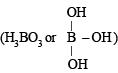is a lewis acid so (a) is
incorrect statement.
The coordination number exhibited by beryllium is 4 and not 6 so statement (b) is incorrect.
Both BeCl2 and AlCl3 exhibit bridged structures in solid state so (c) is correct statement.

QUESTION: 29

Which one of the following reactions of xenon compounds is not feasible?

Solution:

The products of the concerned reaction react each other forming back the reactants.

XeF6 + 3H2O → XeO3+ 6HF .

QUESTION: 30

Which of the following statement is wrong?

Solution:

The ease of for mation an d stability  of hydrides decreases rapidly from NH3 to BiH3. This is evident from their dissociation temperature which decreases from NH3 to BiH3. As we go down the group the size of central atom increases and thus metal-hydrogen bond becomes weaker due to decreased overlap between the large central atom and small hydrogen atom.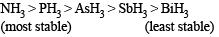QUESTION: 31

Which of the following statements regarding sulphur is incorrect?

Solution:

Oxidation of sulphur varies from – 2 to + 6 in its various compounds

QUESTION: 32

Boron cannot form which one of the following anions?

Solution:

Boron cannot form BF63– due to non-availability of d-orbitals.

QUESTION: 33

The molecule having smallest bond angle is :

Solution:

All the members form volatile halides of the type AX3.  All  halides are pyramidal in shape.  The bond angle decreases on moving  down the group due to decrease in bond pair-bond pair repulsion.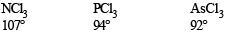QUESTION: 34

Among the following oxoacids, the correct decreasing order of acid strength is:

Solution:

Acidic strength increases as the oxidation number of central atom increases.
Hence acidic strength order is

(+7)         (+5)         (+3)        (+1)
HClO4 > HClO3 > HClO2 > HClO

QUESTION: 35

Which one of the following properties is not shown by NO?

Solution:

Nitric oxide is par amagn etic in th e gaseous state because of the presence of one unpaired electron in its outermost shell.
The electronic configuration of NO is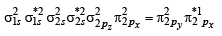QUESTION: 36

The correct statement for the molecule, CsI3 is:

Solution:

CsI3 dissociates as

CsI3 → Cs+ + I3

QUESTION: 37

Which among the following is the most reactive ?

Solution:

ICl

Order of reactivity of halogens
Cl2 > Br2> I2

But, the interhalogen compounds are generally more reactive than halogens (except F2), since the bond between two dissimilar electronegative elements is weaker than the bond between two similar atoms i.e, X – X

QUESTION: 38

Assertion: Nitrogen and oxygen are the main components in the atmosphere but these do not react to form oxides of nitrogen.
Reason: The reaction between nitrogen and oxygen requires high temperature.

Solution:

Nitrogen and oxgen in air do not react to form oxides of nitrogen in atmosphere because the reaction between nitrogen and oxygen  requires high temperature.

QUESTION: 39

Which one has the highest boiling point ?

Solution:

Xe. As we move down the group, the melting and boiling points show a regular increase due to corresponding increase in the magnitude of their van der waal forces of attraction as the size of the atom increases.

QUESTION: 40

The pair in which phosphorous atoms have a formal oxidation state of + 3 is :

Solution:

Phosphorous acids contain P in + 3 oxidation state.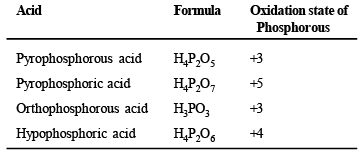QUESTION: 41

The reaction of zinc with dilute and concentrated nitric acid, respectively, produces:

Solution: